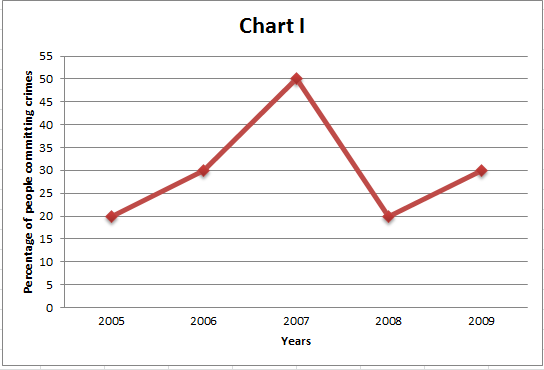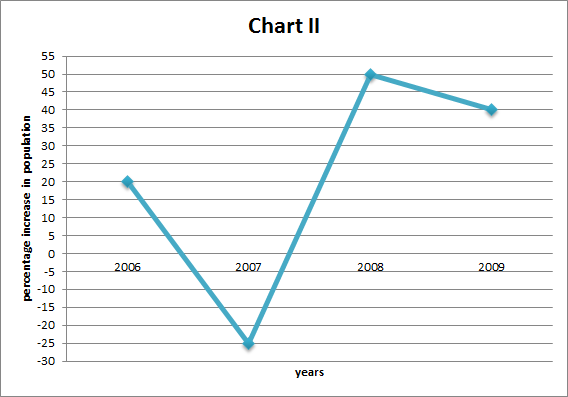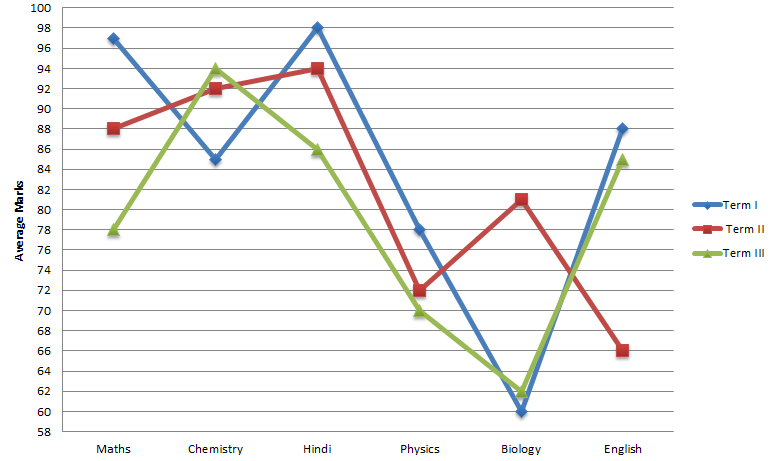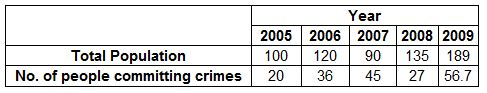# Line and Graph Questions for CAT PDF (Set – 2)

1
3737

Line and Graph Questions for CAT PDF (Set – 2):

Instructions (1 – 4): Refer to the following graphs and answer the given questions:
Graph I gives the percentage of people committing crimes each year in Boselvania and graph II gives the percentage increase in the population of Boselvania each year compared to the previous year.Question 1: In which year among the given years(from 2005-2009) was the number of people that committed crimes the the second lowest?

a) 2005
b) 2006
c) 2007
d) 2008
e) 2009

Question 2: What was the percentage of the population in 2008 compared to 2006?

a) 112.5%
b) 137.5%
c) 125%
d) 100%
e) none of these

Question 3: In which year among the given years (2005-2009) was the population in Boselvania the third highest?

a) 2005
b) 2006
c) 2007
d) 2008
e) none of these

Question 4: What is the ratio of the number of people committing crimes in 2007 compared to 2008?

a) 4:1
b) 5:3
c) 2:1
d) 3:2
e) cannot be determined

Instructions (5 – 8): The following chart gives the average of marks in six subjects scored by 25 students in three different exams conducted in an academic year.The maximum marks of the exam is 100 and a student who scores at least 35 marks is
considered as passed.

Question 5: The number of students that scored more than 80 marks in all the subjects in all the term cannot be more than

a) 16
b) 17
c) 18
d) 19

Question 6: If no student failed in any of the subjects in term III, then what is the maximum number of students who can score full marks in a single subject?

a) 21
b) 22
c) 23
d) 24

Question 7: The number of students who failed in all the subjects in the IInd term cannot be more than?

a) 1
b) 2
c) 3
d) 4

Question 8: What could be the maximum number of students that failed in all the exams in all the three terms?

a) 0
b) 1
c) 2
d) 3

Solutions (1 – 4) :
For ease of calculation let’s assume the number of people in Boselvania in 2005 is 100. In Graph II, the percentage increase in 2006 is 20%. Thus, the population would be 100×1.2 = 120 in 2006. Also, the percentage crimes in Boselvania in 2006 is 30%. Thus, the number of crimes in Boselvania is 120x.3 = 36. In this way, the table can be formed for all the given years.1) Answer (D)
From the table, we can see that the number of people committing crimes was second lowest in 2008

The number of people in 2008 = 135
The number of people in 2006 = 120
Thus, the percentage of population in 2008 compared to 2006 = $$\frac{135}{120}\times100$$% = 112.5%

From the table, we can see that the population was third highest in the year 2006.

Number of crimes in 2007 is 45 and number of crimes in 2008 are 27.
Thus, the required ratio = 45:27 = 5 : 3

Solutions (5 – 8):

The number of students that scored more than 80 in all subjects in all the terms will be limited by the subject having the lowest average which is 60 for Biology in the first term.
The total score of all the sixty students = 60 x 25 = 1500
Thus, the maximum number of students who scored greater than 80 in all the six subjects for all the terms = 1500/80 = 18.75 i.e 18 students.

The number of students scoring 100 will be maximum for the subject having the highest average in term III which is Chemistry.
The total marks scored by all the 25 students in Chemistry in term III = 25 x 94 = 2350
Thus, the maximum number of students that could have scored 100 for Chemistry in term III = 23 But this is not possible as no student should fail.
If 22 students get 100, then the remaining total marks for 3 students is 2350 – 2200 = 150. Thus, the marks can be distributed that all the three students pass. Hence, a maximum of 22 students can score full marks in a single subject.

The number of students failed will be minimum for the subject with the maximum average marks.
For the II term, Hindi has the maximum average marks.
The total marks scored by all the 25 students in Hindi = 25 x 94 = 2350.
If we assume 3 students failed, then the maximum total marks of the remaining 22 students =2350 – 34×3 = 2248.
But since maximum marks for a subject is only 100, this is not possible.
If we assume 2 students failed, then the maximum total marks of the remaining 23 students = 2350 – 34×2 = 2282 which is possible. Thus, the maximum number of students who have failed in all the subjects in the second term = 2